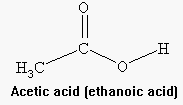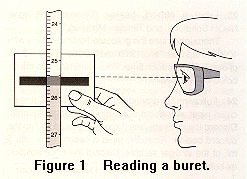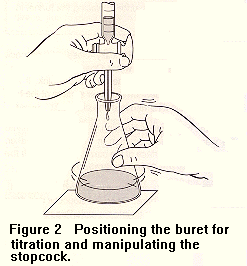CHEMISTRY 122: VINEGAR ANALYSIS

BACKGROUND INFORMATIONVinegar is a sour liquid consisting mainly of acetic acid and water. Acetic acid (CH3COOH or HC2H3O2) is the source of the acidity in vinegar. Acetic acid (ethanoic acid) is an organic acid (carboxylic acid) and is classified as a weak acid.

Vinegar is regulated by the Food and Drug Administration (FDA) and to be legal must have a minimum of 5% acidity. The percent acidity (percent acetic acid) in a sample of vinegar is calculated by the Equation 1.

(Eq. 1)      % acidity = (grams of acetic acid / grams of vinegar) x 100

In this experiment a commercial vinegar sample will be analyzed for its percent acidity by an analytical procedure called titration. Titration is an analytical method for determining the concentration of an acid or base by adding quantities of either the acid or the base of known concentration (standard solution) to a measured volume of the test solution until complete neutralization occurs. In this experiment the gradual addition of a basic solution (NaOH) of known concentration will be gradually added to a known volume of the vinegar solution. The point at which the neutralization is complete is referred to as the endpoint and is observed by the color change in the added indicator, phenolphthalein.

The analysis of vinegar will be accomplished out by titrating a 5.00 mL of sample of vinegar with a solution of NaOH of known molarity, according to Equation 2.

(Eq. 2)          CH3COOH(aq) + NaOH(aq) ---> CH3COONa(aq) + H2O(l)

Because the reaction stoichiometry is one mole of acetic acid reacting with one mole of sodium hydroxide, the number of moles of acetic acid in the portion of the vinegar sample titrated can be found from Equation 3.

(Eq. 3)              moles of acetic acid = (MNaOH)(VNaOH)

MNaOH is the molarity of the sodium hydroxide solution in moles/L and VNaOH is the volume of sodium hydroxide solution in liters.

The grams of acetic acid in the vinegar sample can be calculated by multiplying the moles of acetic acid by the molecular weight of acetic acid (MW). This is shown in Equation 4.

(Eq. 4)               grams of acetic acid = (moles of acetic acid)(MW)

By assuming the density of the vinegar sample to be 1.00 g/mL, the grams of vinegar solution can be calculated by multiplying the volume of vinegar by the density. This is shown in Equation 5.

(Eq. 5)               grams of vinegar = (volume of vinegar)(density of vinegar)

The percent by mass of acetic acid in the vinegar sample can be found from Equation 1.

(Eq. 1)           % acetic acid = (grams of acetic acid / grams of vinegar) x 100

CHEMISTRY 122: VINEGAR ANALYSIS PROCEDURE
 PUT ON YOUR CHEMICAL SPLASH-PROOF SAFETY GOGGLES!

1. Put on the Goggles!
2. Obtain the molarity of the NaOH from the label on the bottle and record the molarity on the data sheet.
3. Clean a buret by rinsing it with tap water and discarding the water. Next, rinse the buret with two 5 mL portions of NaOH standard solution, discarding the NaOH after each rinse.
4. Fill the buret with NaOH standard solution to slightly above the zero mark and set up the buret as demonstrated by the lab instructor.
5. Drain enough NaOH solution from the buret so as to remove all the air bubbles from the tip of the buret and read the level as shown in Figure 1. Record this reading as the initial volume to ± 0.01 mL on the data sheet for NaOH.
6. Repeat steps 2 - 4 for the vinegar buret.

7. Drain approximately 5 mL of the vinegar from the buret into a clean 250 mL Erlenmeyer flask. Record the final volume to ± 0.01 mL on the data sheet for vinegar.
8. To the vinegar sample, add about 20 mL of distilled water and four drops of phenolphthalein indicator solution.
9. As shown in Figure 2, carefully place the flask containing the vinegar and indicator under the buret. Slowly add NaOH from the buret to the flask, while swirling the flask, until the solution in the flask is a faint permanent pink. If you overshoot the end point, the solution will be red and you must discard your results. Record the final volume to ± 0.01 mL on the data sheet for NaOH.

10. Perform a second titration by refilling the NaOH buret and repeating steps 6 - 8.

11. Carry out the calculations as shown on the next page.CHEMISTRY 122: DATA SHEET VINEGAR ANALYSIS Name __________________________ Hood No. _________________ Date _________________

 PUT ON YOUR CHEMICAL SPLASH-PROOF SAFETY GOGGLES!
 Show all calculations.
Molarity of NaOH __________
 TITRATION #1 NaOH VINEGAR Initial Volume Final Volume Volume Used
 TITRATION #2 NaOH VINEGAR

CALCULATIONS

 Moles of acetic acid = (MNaOH)(VNaOH) Note:  VNaOH should be in "Liters"

Grams of acetic acid = (moles of acetic acid)(MW)

Grams of vinegar =(volume of vinegar)(density of vinegar) [NOTE: The density of the vinegar is 1.00 g/mL.]

% acetic acid = (grams of acetic acid / grams of vinegar) x 100

 TITRATION #1 TITRATION #2 Moles of acetic acid Grams of acetic acid % acetic acid in vinegar

AVERAGE % acetic acid in vinegar __________________________